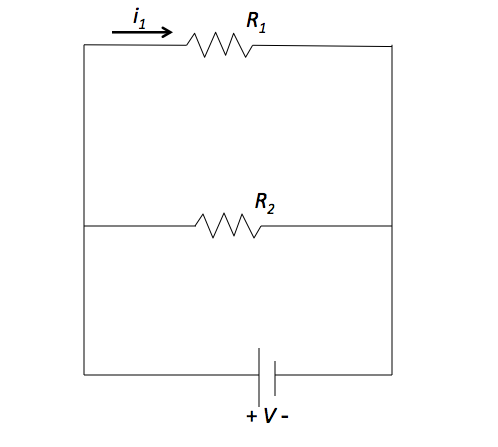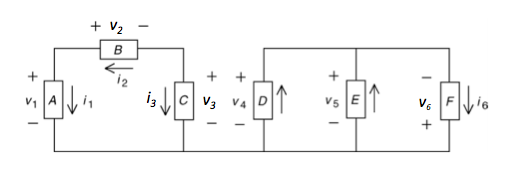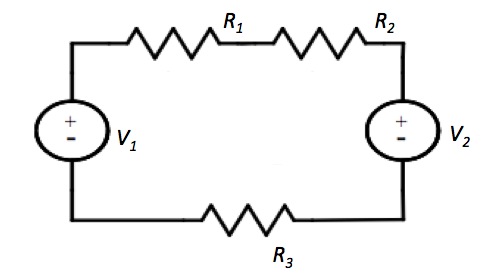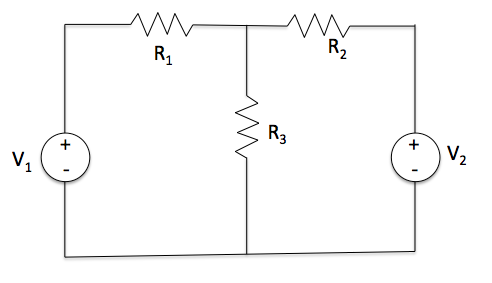Electricity and Magnetism

# Kirchoff's Voltage Law (closed loops)The above circuit consists of resistors and a voltage source. If voltage source is given as $V = 16 \text{ V}$ and the resistances are given as $R_1 = 2 \Omega$ and $R_2 = 4 \Omega,$ what is the current passing through the resistor $R_1?$Consider the circuit shown in the figure above. The voltages and currents are $v_2 = -2 \text{ V}, v_3 = 8 \text{ V}, v_6= 12 \text{ V}, i_3 = i_4 = i_5 = 4 \text{ A}.$ Determine the values of the voltage across the circuit element $E.$The above circuit consists of resistors and voltage sources. The voltages are given as $V_1 = 32 \text{ V}$ and $V_2 = 16 \text{ V},$ and the resistances are given as $R_1 = 2 \Omega, R_2 = 4 \Omega$ and $R_3 = 6 \Omega.$ What is the voltage across the resistor $R_3?$In the above circuit, the voltage source gives $V = 7 \text{ V}.$ When the resistance is given by $R = 2 \Omega,$ what is the voltage $V_R$ across the resistor?The above circuit consists of resistors and voltage sources. The voltage sources are given as $V_1 = 90 \text{ V}$ and $V_2 = 18 \text{ V},$ and the resistances are given as $R_1 = 3 \Omega, R_2 = 6 \Omega$ and $R_3 = 9 \Omega.$ What is the current flow through the resistor $R_3?$

Assume that the ground is at the node under the voltage sources.

×